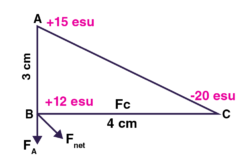# Let ABC is a right angled triangle in which AB = 3 cm and BC = 4 cm and ?ABC=90 o . The three charges +15, +12 and -20 esu are placed on A, B and C respectively. Calculate the force acting on B.The net force on B can be calculated as follows

FNet = √FA2 + FC2

Now calculate FA and FC

FA = (15 Χ 12) / (32)

FA = 20 dyen

FC = (12 Χ 20) / (42)

FC = 15 dyen.

FNet = √FA2 + FC2

FNet = √(20)2 + (15)2

FNet = 25 dyen.